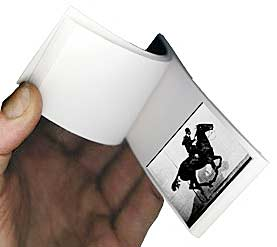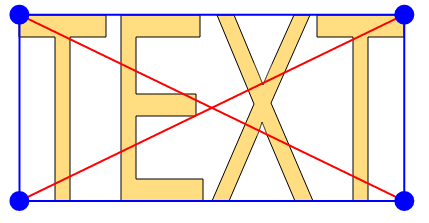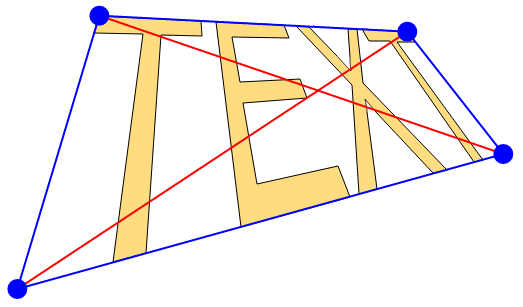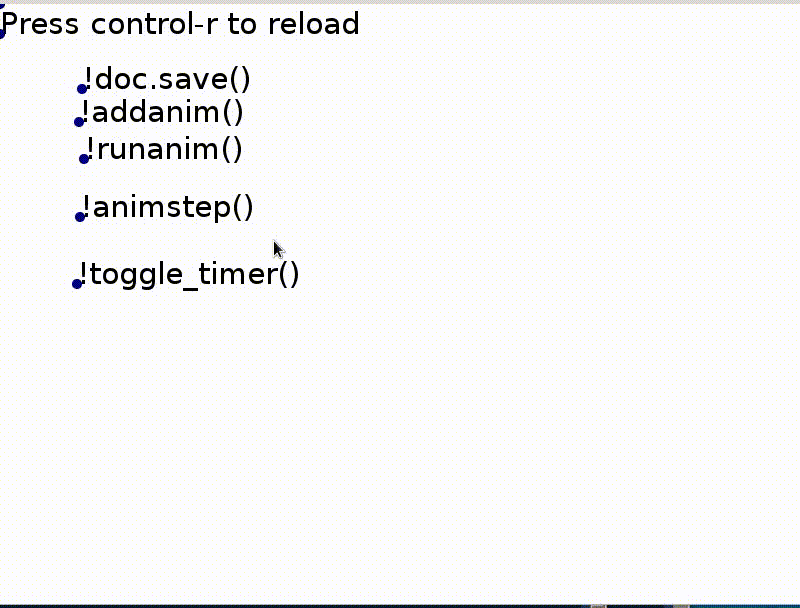# Describing animations

Flipbooks provide the simplest way to animate and the basic principle remain the same with computers.Display a sequence of images fast enough and the eye perceives motion. In this post, I want to look at possible architectures and APIs for an animations library (to potentially be used with Make these slides or plain guitktk).

## Raster image sequence

The first option is to match the flipbook experience exactly. The library user provides an array of images (and possibly a delay between them) and the library displays them in sequence.

Animated gifs essentially work this way. I'm only talking about describing an animation exactly and so won't be looking at rendering optimizations. Something like this [code].

``````show_in_order([image1, image2, image3, image4])
``````

## Vector images

Editing images frame-by-frame is tedious (but still heavily in use today [frames]) but if the images have a vector description (again, skipping over how they are rasterized) then the entire sequence can be replaced by a single image parametrized by time.

``````show_in_order([image(0), image(0.1), image(0.2), image(0.3)])
``````

Or really just something like

``````show_in_order(image, start=0, end=1)
``````

and the library can evaluate the images at run time (or cache them).

``````show_in_order(Node("circle", center=lambda t: (100*t, 100)),
start=0, end=1)
``````## Interpolation

Coming up with expressions like `(100*t, 100)` itself may not be intuitive if the animation becomes complex. Alternatively, we could specify a fixed (non-parametrized) start and ending image (i.e., `image(0.0)` and `image(1.0)`) and interpolate between them to get the rest.

``````show_in_order(interpolate(start_img=Node("circle", center=(0, 100)),
end_img=Node("circle", center=(100, 100))))
``````

Then `image(t)` is defined implicitly as a weighted average of the start and end images.

``````image(t) = start_img * (1-t) + end_img * t
``````

(for `t` from 0 to 1) provided all operators behave as expected. We could pick how many intermediate images we want. For non-linear motion, like an accelerating circle, we'd also want to pick a different interpolation function.

To do that, we can still vary `t` linearly from 0 to 1 but apply another function before getting the image.

``````show_in_order([image(f(0)), image(f(0.1)), image(f(0.2)), image(f(0.3))])
``````

We can pass `f` to `interpolate` and have it replace `image(t)` by `image(f(t))` in all outputs.

## Changes

Instead of an end image, we could just say what has changed

``````interpolate(start_img=Node("circle", center=(0, 100)),
diff={"center": (100, 100)})
``````

If some parts of the image changes a few times, this is helpful to avoid repetition.

## Automatic animation on changes

Based on this idea, we can fire up an animation as part of setters (for properties of objects in the image).

``````circle1 = Node("circle", center=(0, 100))
root.append(circle1)
circle1["center"] = (100, 100)
``````

This is essentially how CSS animations work.

## Affine transformations

For animation that involves movement, instead of changing individual values, a linear transformation could be applied to part or all of the image.

Affine transforms can be represented by a matrix

``````[ a b x
c d y
0 0 1 ]
``````

and is applied to each point (x, y) by multiplying by (x, y, 1) and reading off the first two coordinates.This is what SVG and other systems use. Product of these matrices always gives another matrix of the same shape.

They are powerful enough that they are used almost exclusively in some cases.

### 3d transform and project

(aka perspective transform)

You could also treat the untransformed image as a cardboard cutout placed flat on the screen and apply a 3d affine transform to that cardboard (and project it back onto the screen for display).## Delay

Combining or composing multiple simple animations could mean anything. One way is just to have them side-by-side and play simultaneously.

Another would be to have them play in sequence.

``````anim_sequence = [animation1, animation2, ...]
show_in_sequence(anim_sequence)
``````

If `animation1` has duration `animation1.duration` and so on, and the entire sequence starts at t=0, then what should `anim_sequence(t)` show?

We can calculate partial sums of durations in the sequence and binary search for `t` in this array of values.

• Everything to the left of the index we found should show the last frame of the animation,
• everything to the right should show the first frame, and
• for the animation at the index we should show `anim_sequence[index](t - partial_sums[index])`

Value should be set in increasing value of index.

## Where to put all this information?

Now for the really hard part.

We've talk about how to describe animation but not where to put the information.

Automatic animation on changes is one possible way.

Another is to have separate Animation objects set the values of their targets.

Or we could have these objects be properties of their target. This is what SVG's SMIL (Synchronized Multimedia Integration Language) does.

A final way would be to set the animated object's property values to functions depending on time.

Unfortunately, my library guitktk was setup so this last option is easiest but I think its hard to combine and compose animations this way.

## Current attempt

I'm still quite undecided on just about everything. Currently, I'm describing animations using nodes in the document tree.

``````group: id="animations"
anim: target="drawing.-1.botleft.value"
start=P(100, 100) end=P(300, 100) max_t=1 func=cube_root
anim: target="drawing.-1.botleft.value"
start=P(100, 200) end=P(300, 200) max_t=1
anim: target="drawing.-2.botleft.value"
start=P(100, 250) end=P(300, 250) max_t=1
``````

and values are set each frame by calling `animstep()`.

``````def interpolate(node, t):
return node['start'] * (1 - t) + node['end'] * t

def animstep():
t = cur_time() - doc['animations.start_t']
finished = True
anims = [(node, t) for node in doc['animations']]
for node, _t in anims:
_t = min(node['max_t'], _t) if node['max_t'] is not None else _t
if _t == node['max_t']:
for child in node:
anims.append((child, t - node['max_t']))
else:
finished = False
if "func" in node:
_t = node["func"](_t)
doc[node['target']] = interpolate(node, _t)
return finished
``````To use fewer frames or to better convey an impression, some more tricks can be used because the viewers are humans.

• Motion blur: To suggest fast movement means not only increasing the speed at which positions change but adding some blur to the image in the direction of the motion.
• Smear frames: Some funny combination of characteristics from different timestamps/frames.

For simple animations for UIs and slides, these probably won't come up.

Other topics that might come up but not mentioned here:

• Interpolation for different types: For numbers and vectors, calculating `start * (1-t) + end * t` is straightforward but for things like colours, it can actually get quite interesting.
• Centers and conjugation: We usually want rotations centered around the object, not the coordinate points' origin. The same goes for scaling.

Some stuff that may be worth looking at.

I found this really nice comparison of different javascript animation libraries. Its great for quickly getting an idea of how different APIs work.

Popmotion is the last one on the list. It focused on UI animation and has almost everything discussed here.

## Footnotes

[code] All "source code" before the very end in this post are for a non-existent library and shown purely for discussing potential APIs.

[frames] Even in some of the vector image programs, the software is mainly there to make creating frames more quickly (but still mostly manually) and applying affine transformations.

Its also possible to go the other way around and transform entire parts of a raster image.

## Image sources

Posted on May 8, 2018